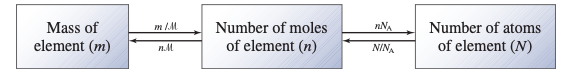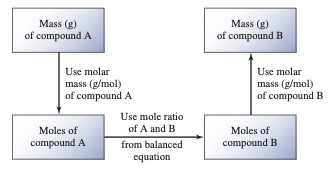# 3.1 Atomic Mass

• Atomic mass is defined as the mass of an atom in atomic mass units, according to international consensus (amu).

• One atomic mass unit is defined as a mass that is one-twelfth that of a carbon-12 atom.

# 3.2 Avogadro’s Number and the Molar Mass of an Element

• Chemists use moles to quantify atoms and molecules.

• The mole (mol) is the amount of a substance in the SI system that has the same number of elementary entities as there are atoms in 12 g (or 0.012 kg) of carbon-12 isotope.# 3.3 Molecular Mass

• The molecular mass (in AMU) is the mass of one molecule.

• The total the atomic masses of each atom in a molecule determines it.

# 3.4 The Mass Spectrometer

• By dislodging an electron from each atom or molecule, collisions between electrons and gaseous atoms produce positive ions.

• As these positive ions (with mass m and charge e) move between the plates, they are propelled by two oppositely charged plates.

# 3.5 Percent Composition of Compounds

• The mass of each element in 1 mole of the compound is divided by the molar mass of the compound and multiplied by 100 percent to get the percent composition.

• Each element in a compound's percent composition by mass is expressed as a percentage by mass.

# 3.6 Experimental Determination of Empirical Formulas

• In addition to the empirical formula, we need to know the compound's approximate molar mass to determine the molecular formula.

# 3.7 Chemical Reactions and Chemical Equations

• They look at what happens to atoms and molecules during a chemical reaction, which is a process that transforms one or more substances into new ones.

• Chemists have devised a standard way to depict chemical reactions using chemical equations to communicate with one another.

• Chemical symbols are used in a chemical equation to show what happens during a reaction.

# 3.8 Amounts of Reactants and Products

• To quantitatively analyze a reaction, we must use our understanding of molar masses and the mole notion.

• The quantitative analysis of reactants and products in a chemical reaction is known as stoichiometry.

• We use moles to compute the amount of product created in a reaction, whether the units for reactants are moles, grams, liters, or some other unit.

• This method is known as the mole method, and it simply means that the stoichiometric coefficients in a chemical equation can be translated into the number of moles of each substance.# 3.9 Limiting Reactants

• The reactants are rarely present in exact stoichiometric amounts, that is, in the proportions suggested by the balanced equation, when a chemist performs a reaction.

• Limiting Reactants 103 quantity of product created relies on how much of this reactant was initially present, the first reactant used up in a reaction is called the limiting reactant.

• Excess reactants are those that are present in greater quantities than are required to react with the limiting reagent.

# 3.10 Reaction Yield

• The theoretical yield of a reaction is determined by the amount of limiting reactant present at the start of the reaction—that is, the amount of product that would result if all of the limiting reactants reacted.

• The theoretical yield, then, is the greatest yield that the balanced equation predicts.

• Almost always, the actual yield, or the amount of product obtained from a reaction, is smaller than the theoretical yield.

• Chemists frequently calculate the percent yield, which is the ratio of the actual yield to the expected yield, to measure how effective a reaction is.